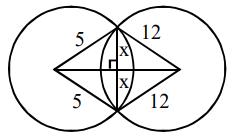# If the angle of intersection at a pointQuestion:

If the angle of intersection at a point where the two circles with radii $5 \mathrm{~cm}$ and $12 \mathrm{~cm}$ intersect is $90^{\circ}$, then the length (in $\mathrm{cm}$ ) of their common chord is :

1. $\frac{60}{13}$

2.  $\frac{120}{13}$

3. $\frac{13}{2}$

4. $\frac{13}{5}$

Correct Option: , 2

Solution:Let length of common chord $=2 x$

$\sqrt{25-x^{2}}+\sqrt{144-x^{2}}=13$

after solving

$x=\frac{12 \times 5}{13}$

$2 x=\frac{120}{13}$